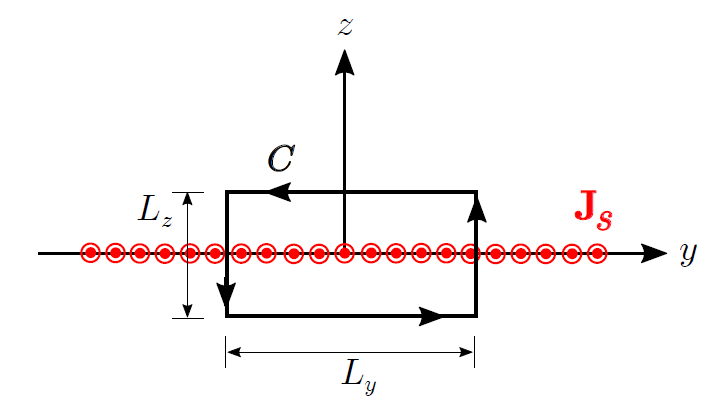# Magnetic Field of an Infinite Current Sheet

We now consider the magnetic field due to an infinite sheet of current, shown in Figure 7.8.1. The solution to this problem is useful as a building block and source of insight in more complex problems, as well as being a useful approximation to some practical problems involving current sheets of finite extent including, for example, microstrip transmission line and ground plane currents in printed circuit boards.Figure 7.8.1: Analysis of the magnetic field due to an infinite thin sheet of current.

The current sheet in Figure 7.8.1 lies in the

plane and the current density is

(units of A/m); i.e., the current is uniformly distributed such that the total current crossing any segment of width

along the

direction is

.

To begin, let’s take stock of what we already know about the answer, which is actually quite a bit. For example, imagine the current sheet as a continuum of thin strips parallel to the

axis and very thin in the

dimension. Each of these strips individually behaves like a straight line current

(units of A). The magnetic field due to each of these strips is determined by a “right-hand rule” – the magnetic field points in the direction of the curled fingers of the right hand when the thumb of the right hand is aligned in the direction of current flow. (Section 7.5). It is apparent from this much that

can have no

component, since the field of each individual strip has no

component. When the magnetic field due to each strip is added to that of all the other strips, the

component of the sum field must be zero due to symmetry. It is also clear from symmetry considerations that the magnitude of

cannot depend on

or

. Summarizing, we have determined that the most general form for

is

, and furthermore, the sign of

must be positive for

and negative for

.

It’s possible to solve this problem by actually summing over the continuum of thin current strips as imagined above.1 However, it’s far easier to use Ampere’s Circuital Law (ACL; Section 7.4). Here’s the relevant form of ACL:

where

is the current enclosed by a closed path

. ACL works for any closed path, but we need one that encloses some current so as to obtain a relationship between

and

. Also, for simplicity, we prefer a path that lies on a constant-coordinate surface. A convenient path in this problem is a rectangle lying in the

plane and centered on the origin, as shown in Figure 7.8.1. We choose the direction of integration to be counter-clockwise from the perspective shown in Figure 7.8.1, which is consistent with the indicated direction of positive

according to the applicable right-hand rule from Stokes’ Theorem. That is, when

is positive (current flowing in the

direction), the current passes through the surface bounded by

in the same direction as the curled fingers of the right hand when the thumb is aligned in the indicated direction of

.

Let us define

to be the width of the rectangular path of integration in the

dimension and

to be the width in the

dimension. In terms of the variables we have defined, the enclosed current is simply

Equation 7.8.1 becomes

Note that

for the vertical sides of the path, since

is

-directed and

on those sides. Therefore, only the horizontal sides contribute to the integral and we have:

Now evaluating the integrals:

Note that all factors of

cancel in the above equation. Furthermore,

due to (1) symmetry between the upper and lower half-spaces and (2) the change in sign between these half-spaces, noted earlier. We use this to eliminate

and solve for

as follows:

yielding

and therefore

Furthermore, note that

is independent of

; for example, the result we just found indicates the same value of

regardless of the value of

. Therefore,

is uniform throughout all space, except for the change of sign corresponding for the field above vs. below the sheet. Summarizing:

The magnetic field intensity due to an infinite sheet of current (Equation 7.8.9) is spatially uniform except for a change of sign corresponding for the field above vs. below the sheet.

## Footnotes

• 1

In fact, this is pretty good thing to try, if for no other reason than to see how much simpler it is to use ACL instead.

Ellingson, Steven W. (2018) Electromagnetics, Vol. 1. Blacksburg, VA: VT Publishing. https://doi.org/10.21061/electromagnetics-vol-1 CC BY-SA 4.0Swipe left and right to change pages.